# Replacement Project Analysis

Replacement Project Analysis is used when the company has to decide whether or not to replace an existing machine or asset. Replacement cost analysis is different from expansion cost analysis for at least two reasons. On the one hand, we have to take into account the sale of the old asset. On the other hand, we have to look at the incremental cash flows.

On this page, we discuss the replacement project analysis formulas and discuss a replacement project analysis example. Using an Excel spreadsheet, we implement a numerical example that illustrates how to apply all the formulas presented.

## Replacement project analysis formulas

First, we need to calculate the initial outlay. The initial outlay has to reflect the sale of the old asset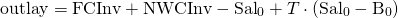where FCInv is the fixed capital investment, NWCInv is the net working capital investment, Sal0 is the revenues from the sale of the old asset, T is the tax rate, and B0 is the book value of the old asset. Clearly, the sale of the old asset reduces the initial outlay.

Next, there’s the incremental operating cash flows. These are the cash flows of the new asset minus the cash flows of the old asset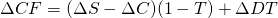where S stands for sales, C stands for cash operating costs, b stands for depreciation expense, and D is the tax rate.

Finally, we need to calculate the terminal year non-operating cash flow (TNOCF)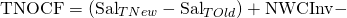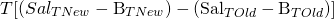## Replacement project analysis example

Next, let’s consider an Example in Excel. The following table shows the implementation of an example. We implement the above formulas to calculate all the cash flows and then calculate the IRR.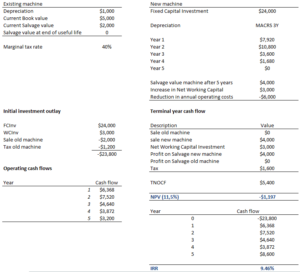The Excel spreadsheet used to create the example can be downloaded at the bottom of the page.

## Summary

We discussed the replacement cost analysis method. It can be used to determine whether or not it makes sense to replace an existing asset with a better one.